# Discrete Mathematics Lecture 20 Sequence Series Sequence Sequence

• Slides: 57Discrete Mathematics Lecture # 20 Sequence & SeriesSequenceSequence A sequence is just a list of elements usually written in a row. 1. 1, 2, 3, 4, 5, … 2. 4, 8, 12, 16, 20, … 3. 2, 4, 8, 16, 32, … 4. 1, 1/2, 1/3, 1/4, 1/5, … 5. 1, 4, 9, 16, 25, … 6. 1, -1, …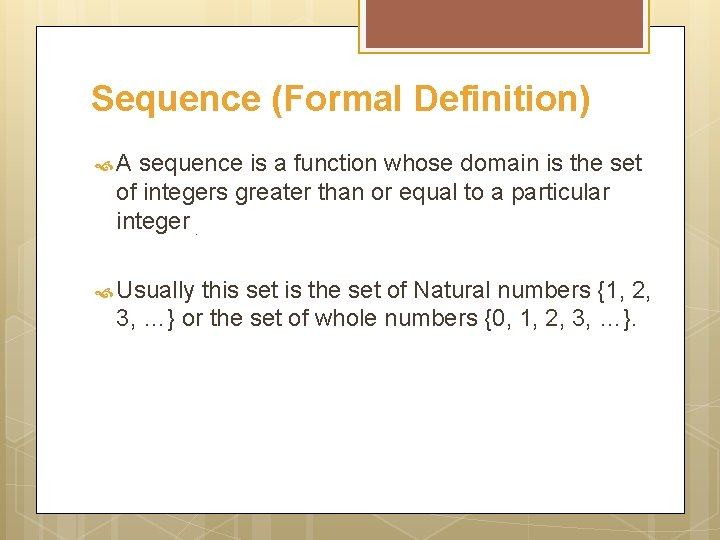Sequence (Formal Definition) A sequence is a function whose domain is the set of integers greater than or equal to a particular integer. Usually this set is the set of Natural numbers {1, 2, 3, …} or the set of whole numbers {0, 1, 2, 3, …}.Sequence Notation We use the notation an to denote the image of the integer n, and call it a term of the sequence. Thus a 1, a 2, a 3, a 4 …, an, … represent the terms of a sequence defined on the set of natural numbers N. Note that a sequence is described by listing the terms of the sequence in order of increasing subscripts.Finding Terms of Sequence using Explicit Formula An explicit formula or general formula for a sequence is a rule that shows how the values of ak depends on k.Example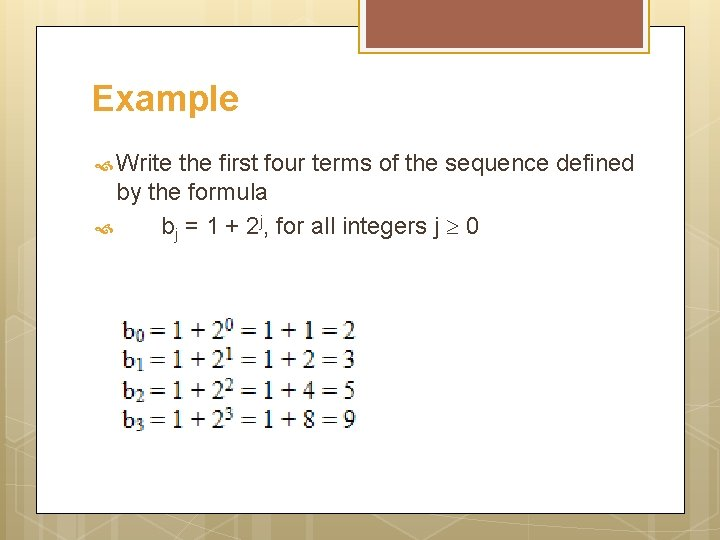Example Write the first four terms of the sequence defined by the formula bj = 1 + 2 j, for all integers j 0Example Compute the first six terms of the sequence defined by the formula Cn = 1+ (-1) n for all integers n 0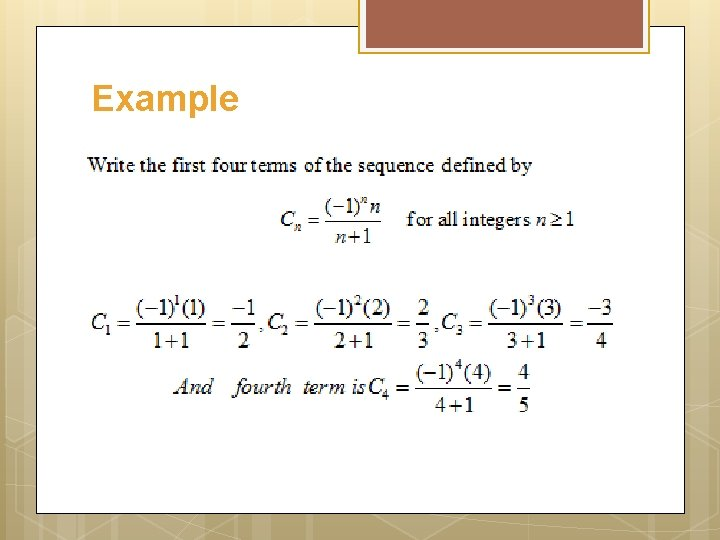Example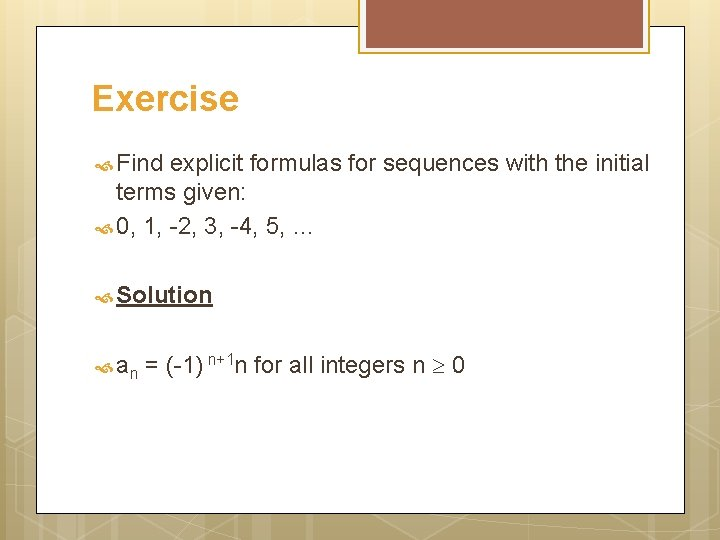Exercise Find explicit formulas for sequences with the initial terms given: 0, 1, -2, 3, -4, 5, … Solution an = (-1) n+1 n for all integers n 0Exercise Solution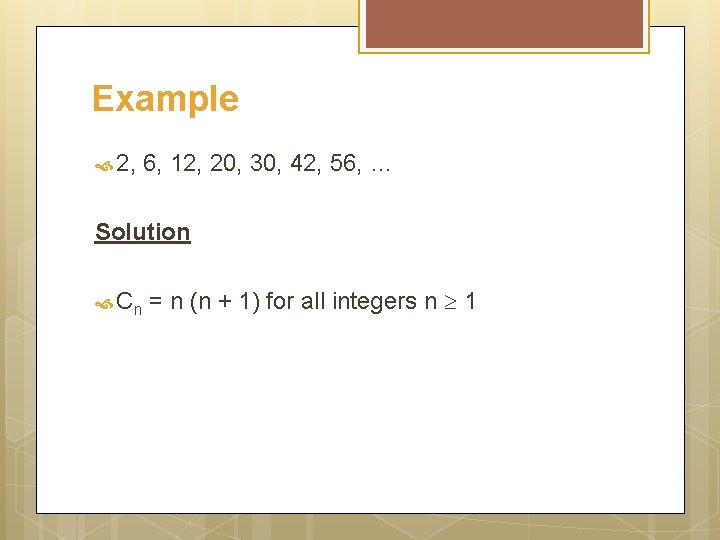Example 2, 6, 12, 20, 30, 42, 56, … Solution Cn = n (n + 1) for all integers n 1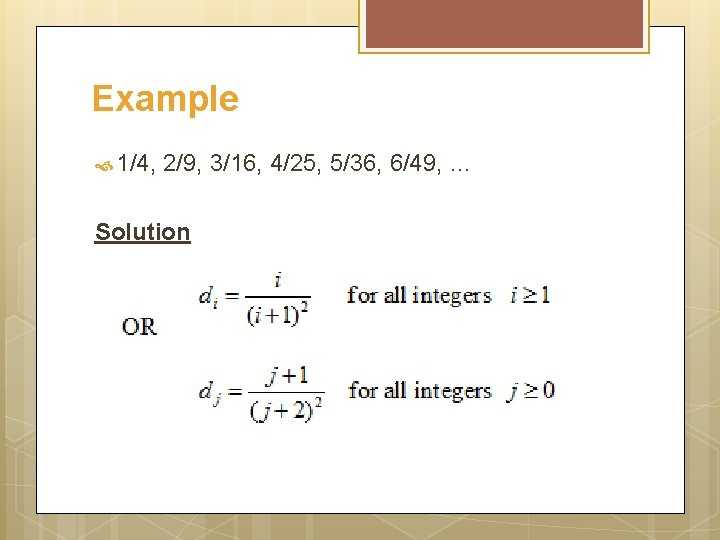Example 1/4, 2/9, 3/16, 4/25, 5/36, 6/49, … Solution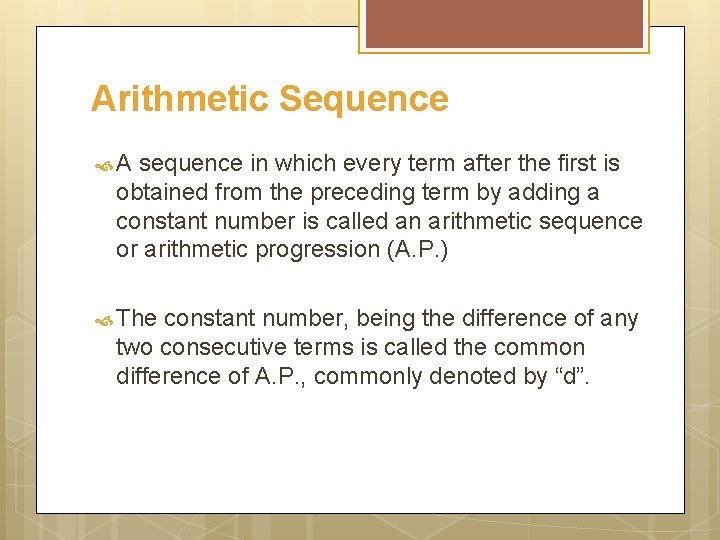Arithmetic Sequence A sequence in which every term after the first is obtained from the preceding term by adding a constant number is called an arithmetic sequence or arithmetic progression (A. P. ) The constant number, being the difference of any two consecutive terms is called the common difference of A. P. , commonly denoted by “d”.Example 5, 9, 13, 17, … (common difference = 4) 0, -5, -10, -15, … (common difference = -5) x + a, x + 3 a, x + 5 a, … (common difference = 2 a)General term of an arithmetic sequence: Let a be the first term and d be the common difference of an arithmetic sequence. Then the sequence is a, a+d, a+2 d, a+3 d, … If ai, for i 1, represents the terms of the sequence then a 1 = first term = a + (1 -1) d a 2 = second term = a + d = a + (2 -1) d a 3 = third term = a + 2 d = a + (3 -1) d By symmetry an = nth term = a + (n - 1)d for all integers n 1.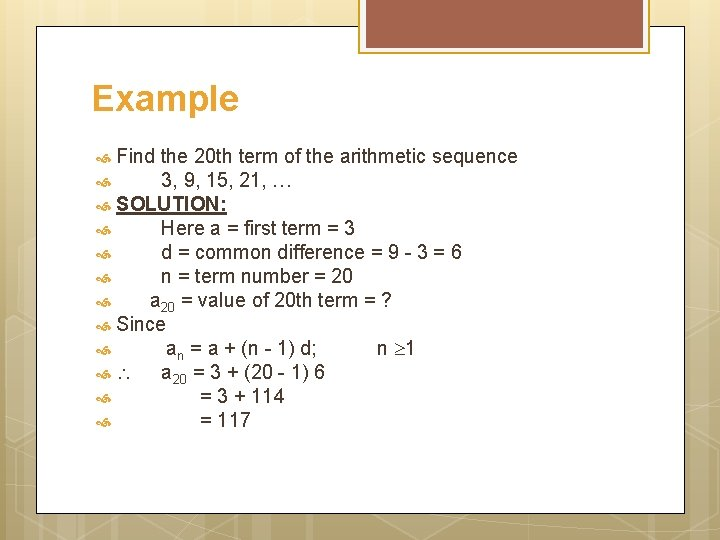Example Find the 20 th term of the arithmetic sequence 3, 9, 15, 21, … SOLUTION: Here a = first term = 3 d = common difference = 9 - 3 = 6 n = term number = 20 a 20 = value of 20 th term = ? Since an = a + (n - 1) d; n 1 a 20 = 3 + (20 - 1) 6 = 3 + 114 = 117Example Which term of the arithmetic sequence 4, 1, -2, …, is -77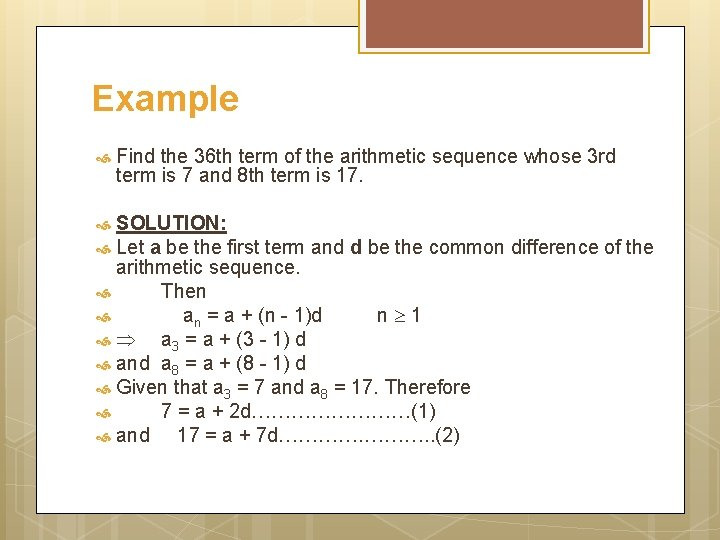Example Find the 36 th term of the arithmetic sequence whose 3 rd term is 7 and 8 th term is 17. SOLUTION: Let a be the first term and d be the common difference of the arithmetic sequence. Then an = a + (n - 1)d n 1 a 3 = a + (3 - 1) d and a 8 = a + (8 - 1) d Given that a 3 = 7 and a 8 = 17. Therefore 7 = a + 2 d…………(1) and 17 = a + 7 d…………. . (2)Example (contd. ) Subtracting (1) from (2), we get, 10 = 5 d d = 2 Substituting d = 2 in (1) we have 7 = a + 2(2) which gives a = 3 Thus, an = a + (n - 1) d an = 3 + (n - 1) 2 (using values of a and d) Hence the value of 36 th term is a 36 = 3 + (36 - 1) 2 = 3 + 70 = 73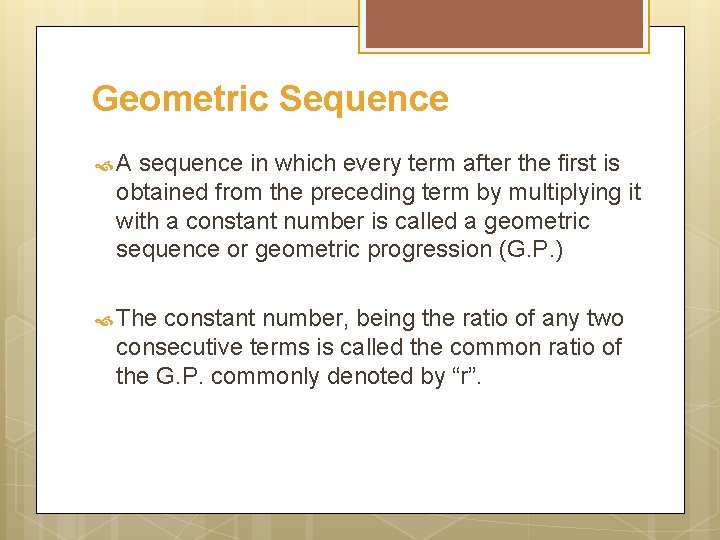Geometric Sequence A sequence in which every term after the first is obtained from the preceding term by multiplying it with a constant number is called a geometric sequence or geometric progression (G. P. ) The constant number, being the ratio of any two consecutive terms is called the common ratio of the G. P. commonly denoted by “r”.Example 1, 2, 4, 8, 16, … (common ratio = 2) 3, - 3/2, 3/4, - 3/8, … (common ratio = - 1/2) 0. 1, 0. 01, 0. 0001, … (common ratio = 0. 1 = 1/10)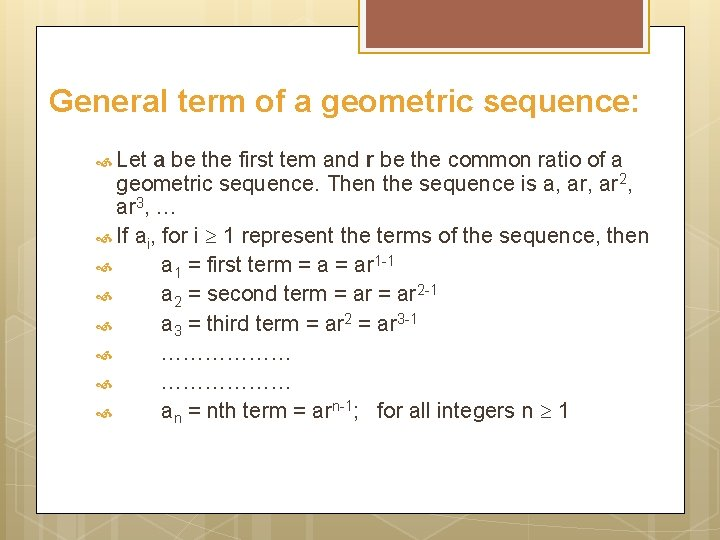General term of a geometric sequence: Let a be the first tem and r be the common ratio of a geometric sequence. Then the sequence is a, ar 2, ar 3, … If ai, for i 1 represent the terms of the sequence, then a 1 = first term = ar 1 -1 a 2 = second term = ar 2 -1 a 3 = third term = ar 2 = ar 3 -1 ……………… an = nth term = arn-1; for all integers n 1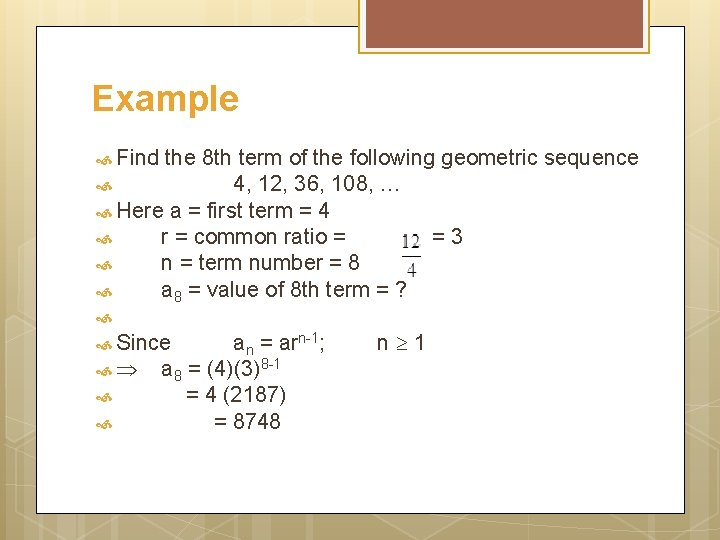Example Find the 8 th term of the following geometric sequence 4, 12, 36, 108, … Here a = first term = 4 r = common ratio = =3 n = term number = 8 a 8 = value of 8 th term = ? Since an = arn-1; a 8 = (4)(3)8 -1 = 4 (2187) = 8748 n 1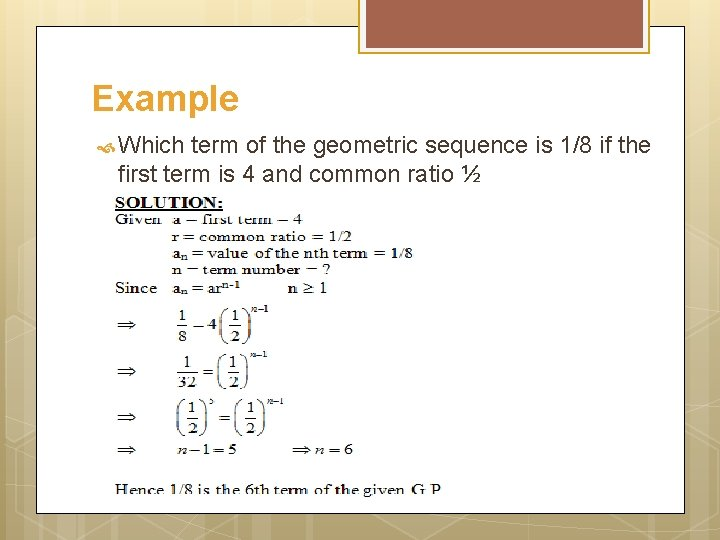Example Which term of the geometric sequence is 1/8 if the first term is 4 and common ratio ½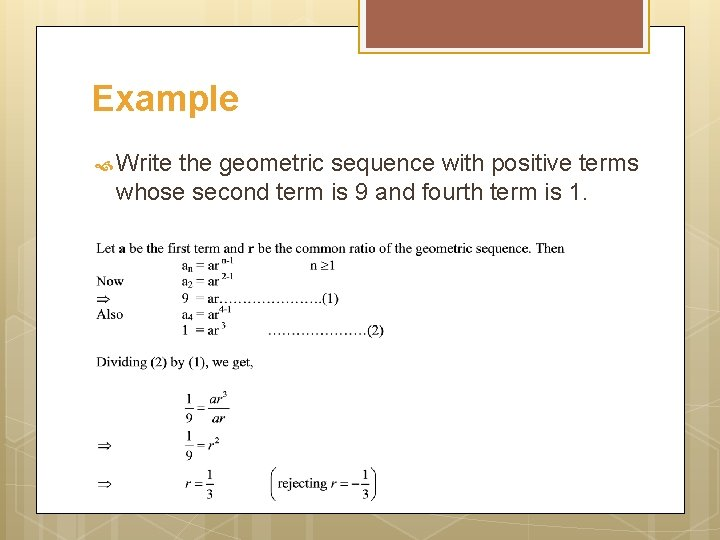Example Write the geometric sequence with positive terms whose second term is 9 and fourth term is 1.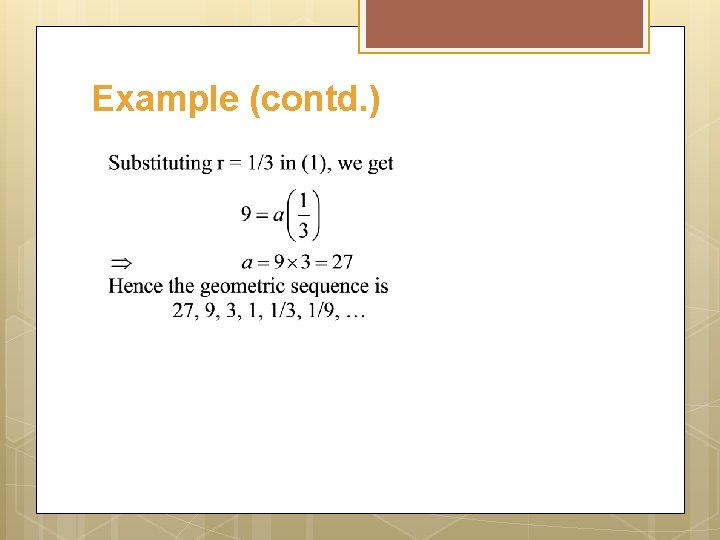Example (contd. )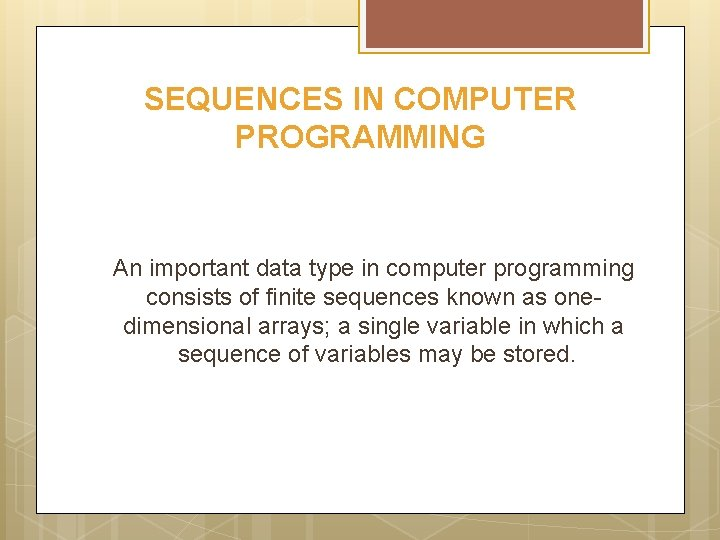SEQUENCES IN COMPUTER PROGRAMMING An important data type in computer programming consists of finite sequences known as onedimensional arrays; a single variable in which a sequence of variables may be stored.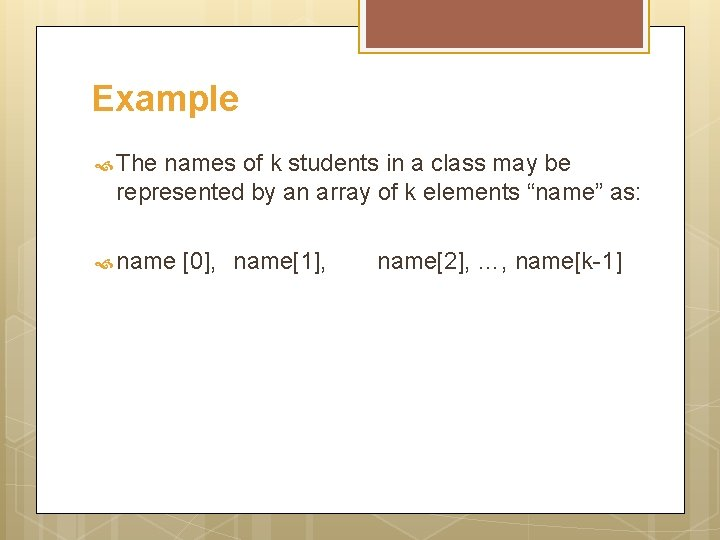Example The names of k students in a class may be represented by an array of k elements “name” as: name , name, name, …, name[k-1]SeriesSeriesSummation Notation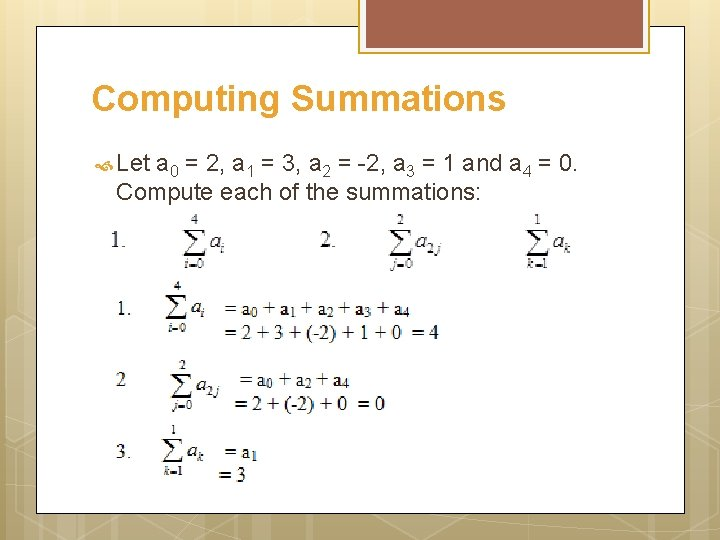Computing Summations Let a 0 = 2, a 1 = 3, a 2 = -2, a 3 = 1 and a 4 = 0. Compute each of the summations:Exercise Compute the summations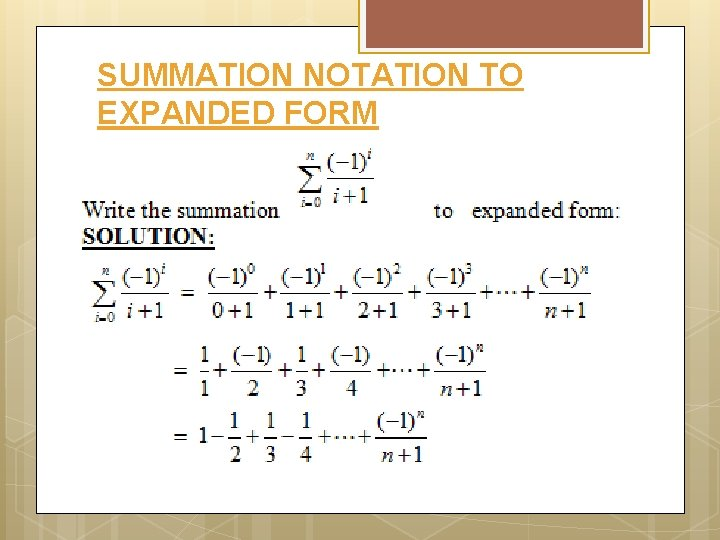SUMMATION NOTATION TO EXPANDED FORMEXPANDED FORM TO SUMMATION NOTATION Solution We find the kth term of the series. The numerators forms an arithmetic sequence 1, 2, 3, …, n+1, in which a = first term = 1 & d = common difference = 1 ak = a + (k - 1)d = 1 + (k - 1) (1) = 1 + k - 1 = kTRANSFORMING A SUM BY A CHANGE OF VARIABLE: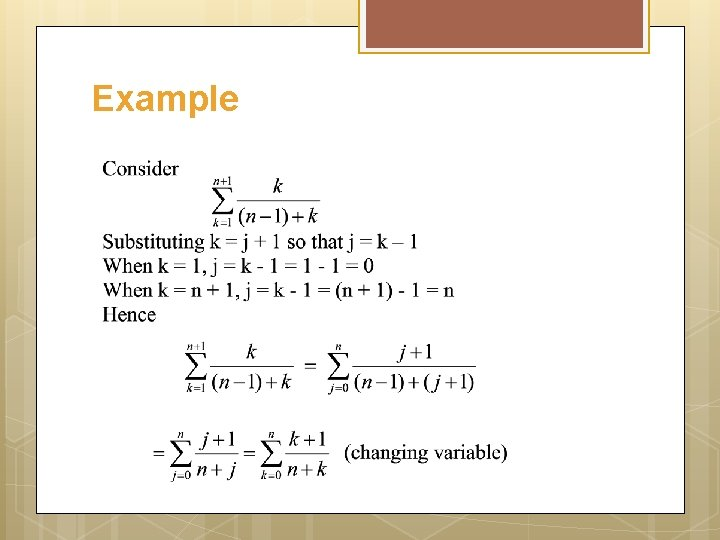Example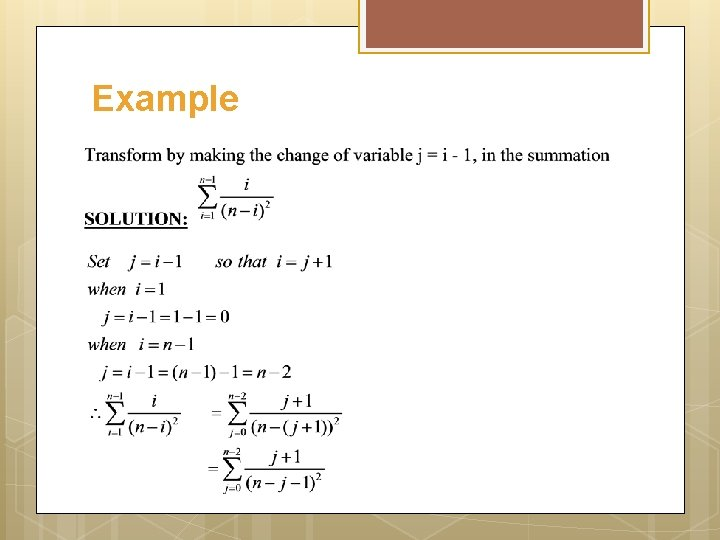Example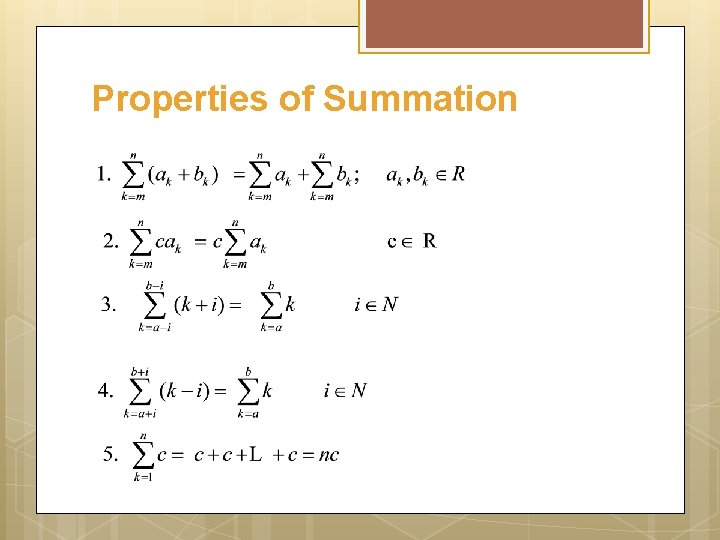Properties of SummationExample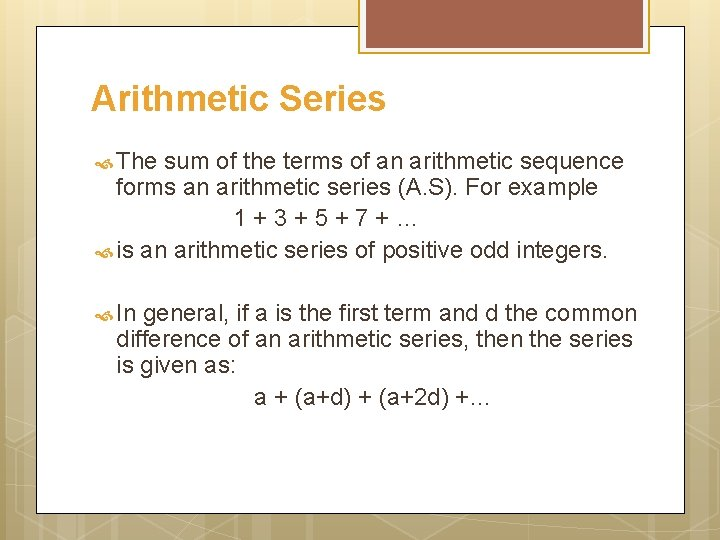Arithmetic Series The sum of the terms of an arithmetic sequence forms an arithmetic series (A. S). For example 1+3+5+7+… is an arithmetic series of positive odd integers. In general, if a is the first term and d the common difference of an arithmetic series, then the series is given as: a + (a+d) + (a+2 d) +…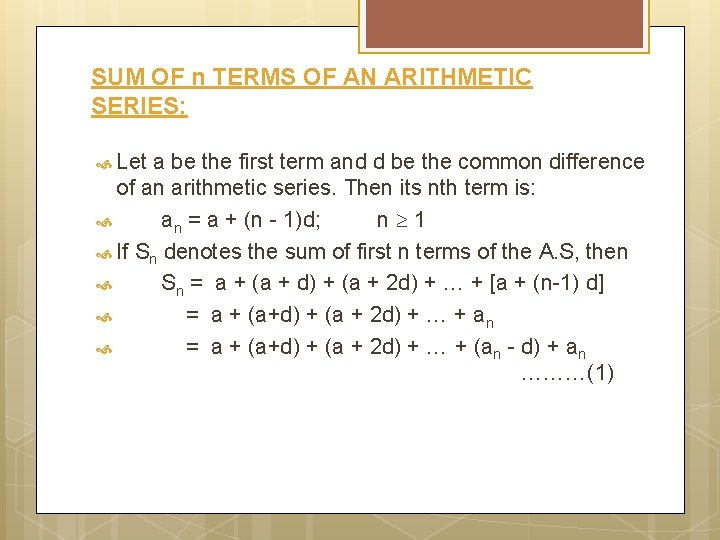SUM OF n TERMS OF AN ARITHMETIC SERIES: Let a be the first term and d be the common difference of an arithmetic series. Then its nth term is: an = a + (n - 1)d; n 1 If Sn denotes the sum of first n terms of the A. S, then Sn = a + (a + d) + (a + 2 d) + … + [a + (n-1) d] = a + (a+d) + (a + 2 d) + … + an = a + (a+d) + (a + 2 d) + … + (an - d) + an ………(1)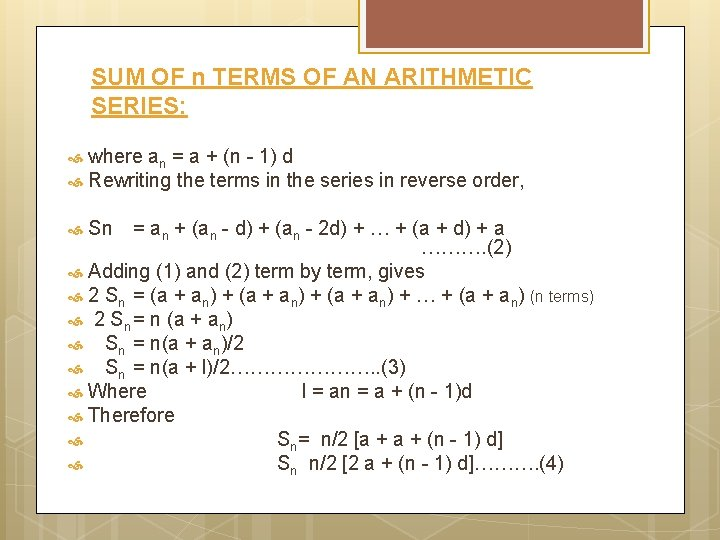SUM OF n TERMS OF AN ARITHMETIC SERIES: where an = a + (n - 1) d Rewriting the terms in the series in reverse order, = an + (an - d) + (an - 2 d) + … + (a + d) + a ………. (2) Adding (1) and (2) term by term, gives 2 Sn = (a + an) + … + (a + an) (n terms) 2 Sn = n (a + an) Sn = n(a + an)/2 Sn = n(a + l)/2…………………. . (3) Where l = an = a + (n - 1)d Therefore Sn= n/2 [a + (n - 1) d] Sn n/2 [2 a + (n - 1) d]………. (4) Sn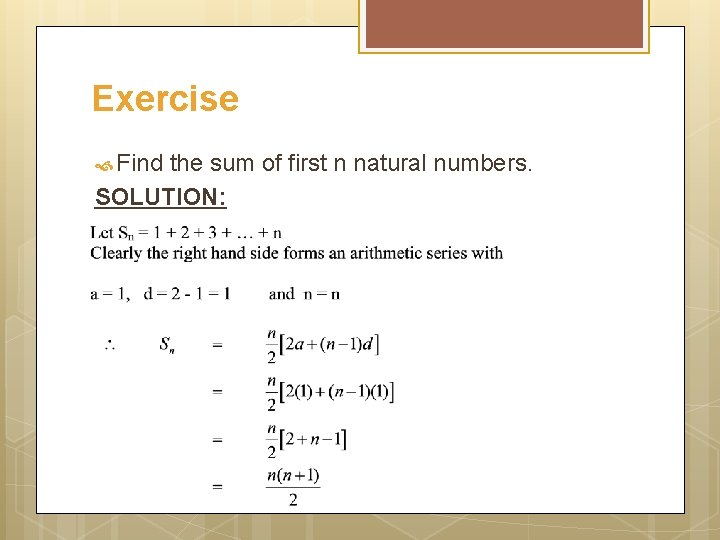Exercise Find the sum of first n natural numbers. SOLUTION:Exercise Find the sum of all two digit positive integers which are neither divisible by 5 nor by 2. SOLUTION: The series to be summed is: 11 + 13 + 17 + 19 + 21 + 23 + 27 + 29 + … + 91 + 93 + 97 + 99 which is not an arithmetic series. If we make group of four terms we get (11 + 13 + 17 + 19) + (21 + 23 + 27 + 29) + (31 + 33 + 37 + 39) + … + (91 + 93 + 97 + 99) = 60 + 100 + 140 + … + 380 which now forms an arithmetic series in which a = 60; d = 100 - 60 = 40 and l = an = 380ExerciseGeometric Series The sum of the terms of a geometric sequence forms a geometric series (G. S. ). For example 1 + 2 + 4 + 8 + 16 + … is geometric series. In general, if a is the first term and r the common ratio of a geometric series, then the series is given as: a + ar 2 + ar 3 + …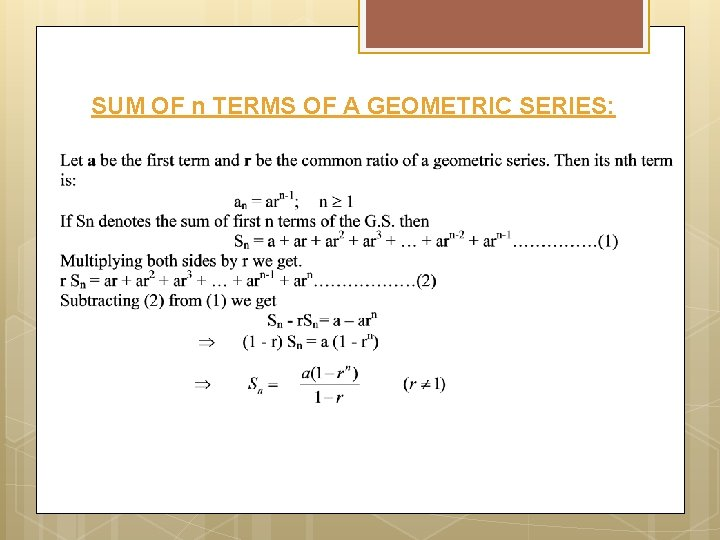SUM OF n TERMS OF A GEOMETRIC SERIES: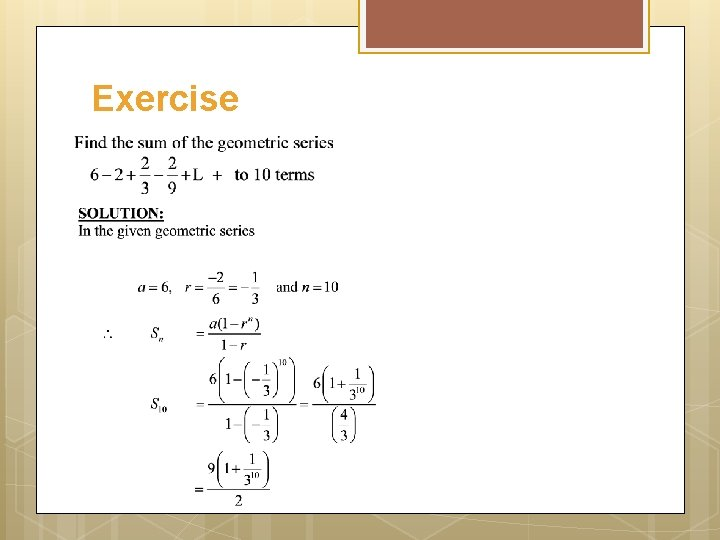Exercise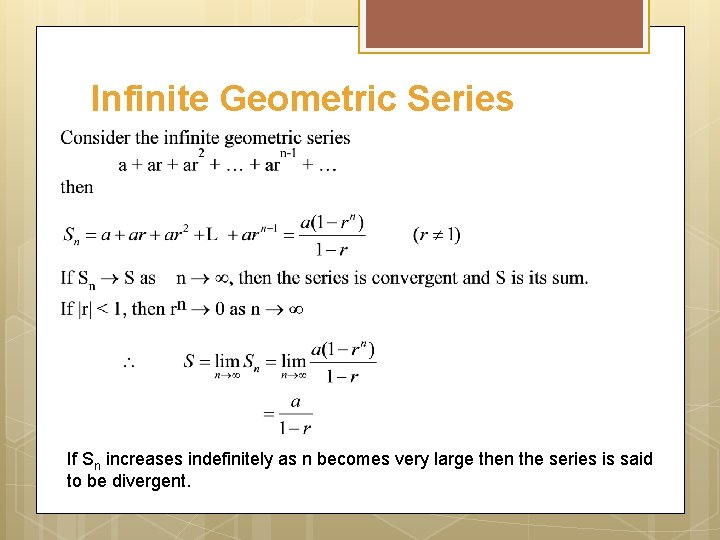Infinite Geometric Series If Sn increases indefinitely as n becomes very large then the series is said to be divergent.Exercise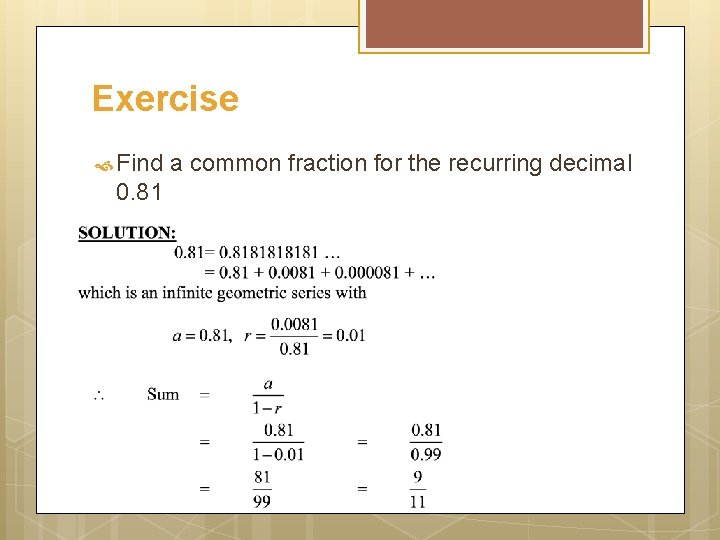Exercise Find 0. 81 a common fraction for the recurring decimalImportant SumsExample Sum to n terms the series 1 5+5 11+9 17+…